# What is Statistics?

Thirumala Reddy

### Last Updated

December 16, 2022

Seclect Blog CategorySTATISTICS

Statistics is the science of collecting, organizing, and analyzing data. Statistics are helpful in better decision-making. It is of 2 types

1)Descriptive Statistics

2)Inferential Statistics

Define Data?

Data is nothing but the Facts & Pieces of information that can be measured.

1)Descriptive Statistics

Descriptive statistics are nothing but Organizing the data, Summarizing the data, and Presenting the data in to an Informative Way.

-->Descriptive statistics focus on describing the visible characteristics of a dataset (a population or sample).

2)Inferential Statistics

It is a technique wherein we use the data that we have measured to form conclusions.

-->Inferential statistics make predictions about a larger dataset, based on a sample of those data.

Population & Sample:-

-->A population is the entire group that you want to draw conclusions about. The population is denoted by N

-->A sample is the specific group that you will collect data from. The size of the sample(n) is always less than the total size of the population(N). The sample is denoted by n.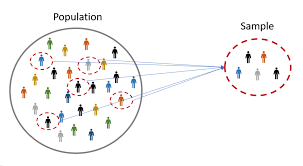Different Sampling Techniques:-

1)Simple Random Sampling:-

In Simple Random Sampling, every number of the population(N) has an equal chance of being selected for your sample(n).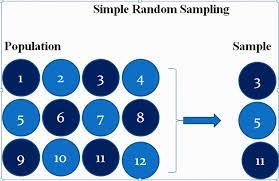2)Stratified Sampling:-
In Stratified Sampling, the Population(N) is split into non-overlapping groups
ex:- The Gender data is divided into 2 groups Male & Female.
3)Systematic Sampling:-
Systematic Sampling is nothing but the from a population N we select every nth individual.

ex:- I am Conducting a survey on Covid in the mall, I will select every 10th person I see for the survey, this is nothing but Systematic Sampling.

4)Convenience Sampling:-
Let's consider that I am doing a survey relating to a specific topic for ex Library used by  Data scientists, the survey is conducted on people who are data scientists. This process is nothing but Convenience Sampling.
Variable: -
A variable is a property that can take on any value.
--> There are two kinds of variables
1)Quantitative Variable
Quantitative variables can be measured numerically i.e. we can perform mathematical operations like add, subtract, multiply, divide, etc. on Quantitative Variable
e.g. height, weight, or age
2)Qualitative/Categorical Variable
Categorical variables are those variables in which the data represent groups. On Qualitative variables, we can perform rankings, classifications, etc.
There are two types of quantitative variables:
1)Discrete  2)continuous.

### Discrete

Quantitative discrete variables are variables that take values that can be countable and have a finite number of possibilities. The values are mostly integers but not always. Some examples of discrete variables are

• Number of children per family
• Number of students in a class
• Number of citizens of a country

#### Continuous

Quantitative continuous variables are variables that take values that are not countable. For example:

• Age
• Weight
• Height
There are 4 types of Measured Variable:-
1)Nominal Data
2)Ordinal Data
3)Interval Data
4)Ratio Data
Nominal data: -
Nominal data is nothing but categorical data. For example, for preferred mode of transportation, we have the categories of car, bus, train, etc.
Ordinal Data: -
In ordinal data, the order of the data matters but value does not matter.
In the above figure, we can say that the order of the data matters but not value i.e. quantity of the data.
Interval data:-
In Interval Data Order of the data matters, and the value of the data matters. Natural Zero is not present in Interval Data
-->Interval data also called an integer, is defined as a data type that is measured along a scale, in which each point is placed at an equal distance from one another.
From the above figure we can observe in the Time column each point is differ by 15min.
Ratio Data: -
Ratio data is nothing but it classifies the data into categories and ranks the data.
Frequency Distribution: -
Frequency Distribution is nothing but the value count of the elements.
Bar Graph: -
The bar Graph is drawn on the basis of Frequency Value. Bar graph works with discrete data. The bar graph is a graph that represents the categorical data with rectangular bars. The bars can be plotted as any one of the types vertically or horizontally.
Histogram: -
Histogram works with continuous value. Similar in appearance to a bar graph.
Arithmetic Mean for Population and sample:-
Mean is nothing but the average. The mean of the Population is given by the symbol
μ. The mean of the sample is given by X̄.

Sample Mean = ∑Xin =(x1+x2+x3+⋯+xn)n

Where,

• ∑xi = sum of values of data
• n = number of values of data
Population Mean (μ) = ∑X / N
∑X is the sum of data in X
N is the count of data in X.
Central Tendency: -
It refers to the measure used to determine the center of the distribution of data. The central tendency measures are Mean, Median, and Mode.
-->Mean is nothing but the average that we discussed above. Mean will be affected by the outliers.
-->Median is nothing the middle value of the data. The median won't get affected by the outliers present in the data.
for example:- consider the data 1,3,4,2,5,6,7,8,0
STEP1:- Sort the data in ascending order
0,1,2,3,4,5,6,7,8
STEP2:- Find out the middle value
The Median is 4.
-->Mode is nothing but the most repeated value in the data. Generally, the mode is used for replacing missing categorical data present in the data.
For example: Consider the data 1,2,4,5,6,7,5,8,5,3,1,2,5,4,5,6,8,9,0,1,3,5
Mode=Most repeated number=5
A measure of Dispersion:-
Dispersion is nothing but the spread of the data.  It says how distributions are different from one another.
-->We can find the spread of the data in 2 ways
1)Variance
2)Standard Deviation
1)Variance:-
Variance is of 2 types i.e. population variance & sample variance.
-->The formula to calculate sample variance is
-->Sample variance is divided by n-1 instead of N because out of the experimentation done by taking different samples from the population and calculating the variance of the sample collected they observed the variance of population and sample varying a lot. In order to overcome this sample variance is divided by n-1. n-1 is performed better when compared to n-2,n-3 .. etc.
2)Standard deviation:-
Standard deviation is nothing but the square root of the variance.
-->We probably prefer the standard deviation to represent the distribution of the data.
-->When the standard deviation is high, we can say that the data is more dispersed. Whenever the standard deviation is low we can conclude that the data is not more dispersed.
Five-number summary:-
Five number summary is used to summarize the data.
-->By using this 5-number summary we will construct Box Plot. A Box plot is nothing but a visualization way to find out the outliers. In 5 number summary, we calculate
1)Minimum
2)First Quartile(Q1)
3)Median
4)Third Quartile(Q3)
5)Maximum
-->After calculating all 5 values we will calculate the lower fence & upper fence.
-->If the data points are below the lower fence & above the upper fence, then those data points are treated as outliers.
IQR=Inter Quartile Range=Q3 - Q1
Upper Fence=Q3 + 1.5*IQR
Lower Fence=Q1 - 1.5*IQR
-->The box plot is constructed below
Let's discuss some of the distributions
Gaussian/Normal Distribution: -
Gaussian/Normal Distribution looks like a bell curve. In this distribution generally, the center is the mean, median, and mode of the data. The area towards the left side of the central line is similar to the area towards the right side of the central line.
-->Some of the examples of data that follow normal distribution are height, weight, IRIS Dataset etc.
Chebyshev's Inequality:-
If the variable belongs to the Gaussian Distribution then we can say the distribution follows Empirical Rule.
-->If a variable does not belong to Gaussian Distribution, then use Chebyshev's Inequality principle to understand the distribution of data.
--> Within the 1.5 Standard deviations, 56% of data is present. Within the 2 Standard deviations, 75% of data is present. Within the 3 Standard deviations, 89% of data was present and within the 4 Standard deviations, 94% of data was present.
Z-Score:-
Z-Score helps us to find out the value is how much the standard deviation is away from the mean. If we get the z-score value as positive(+ve) then we can say the datapoint is present towards the right side similarly vice-versa.
-->If we apply the Z-Score formula to every data point present in the distribution. After applying Z-Score the distribution is converted into the mean=0 & Standard deviation=1
-->The distribution with mean=0, Standard deviation=1 then this distribution is called STANDARD NORMAL DISTRIBUTION
Standardization:-

The process of converting the distribution with mean=0 & standard deviation=1 is called Standardization. In standardization internally Z-Score formula is applied.

Normalization:-
Normalization gives us the process where we can define the lower bound & upper bound and convert the distribution between the lower bound & upper bound.
-->MinMax scaler is used to convert the data into normalization.
Value:-
P value is nothing but the probability for the null hypothesis to be true.
--> Let's consider a space bar of a keyboard, we mostly touch the middle of the space bar. If the P=0.8 i.e. out 0f 100 touches of the spacebar, 80 times touched in the middle of the spacebar.

--> Let's understand the hypothesis testing, confidence interval, and significance value by taking tossing a coin as an example
-->We need to test whether the coin is a fair coin or not by performing 100 tosses.
A coin is said to be fair, if we get P(H)=0.5, P(T)=0.5

Hypothesis Testing:-
1)Null Hypothesis:- Coin if fair
2)Alternate Hypothesis:- Coin is unfair
3)Experiment
4)Reject or Accept the null hypothesis
If the significance value is 0.05, usually significance value is given by domain experts. For a significance value of 0.05, the confidence interval is 95%.
-->Out of 100 times tossed if we get 80 Heads then according to a 95% confidence interval. The coin is fair. So, accept the null hypothesis & reject the alternate hypothesis.
PERFORMANCE METRICS:-
Performance metrics are used to find out how well our Model is working

1)Confusion Matrix:-
A confusion matrix is used for evaluating the results of a classification machine learning model.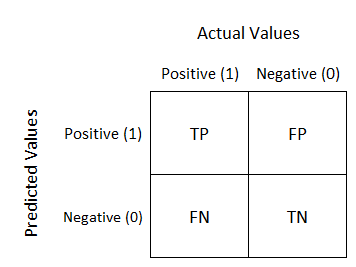where,
TN(True Negatives) - model predicts negative outcomes and the real/known outcome is also negative
TP(True Positives) - The model predicts a positive outcome and the real outcome is also positive
FN(False Negatives) - model predicts a negative outcome but the known outcome is positive
FP(False Positives) - model predicts a positive outcome but the known outcome is negative
2)Type 1 Error:-
Type 1 Error is nothing but we reject the null hypothesis when in reality it is true. Type 1 Error is called FPR
3)Type 2 Error:-
Type 2 Error is nothing but we accept the null hypothesis when in reality it is False. Type 2 Error is called FNR.
4)ACCURACY:--
Accuracy is the ratio of the Number of correct predictions to the Total number of predictions
-->Generally the result of accuracy is taken into consideration for Balanced data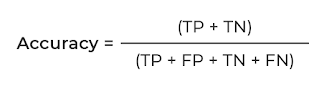5)PRECISION:--
Precision can be defined as out of total actual predicted positive values, how many values are actually positive is called Precision.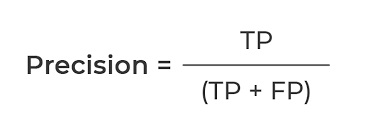• Whenever FP is more important to reduce the use of Precision

eg:-In Spam classification, if we got spam mail it should be identified as spam & in spam classification, we should concentrate on reducing FP i.e. even though the mail we got is not spam but if our algorithm detects it as spam, then we going to miss our important emails .so in order to avoid this case we should concentrate on reducing FP

6)RECALL:--
Recall can be defined as out of total actual positive values, how many values we correctly predicted as positive is called Recall.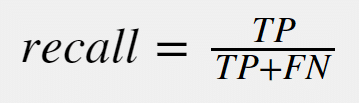• Whenever FN is more important to reduce use Recall

eg:-In classifying a person whether we have cancer or not FN is more important to reduce. If our model predicts that a person doesn't have cancer even though he has cancer this leads to an increase of cancer cells in his body & affects his health.

7)F-BETA:--
The F-beta score is nothing but the weighted harmonic mean of precision and recall.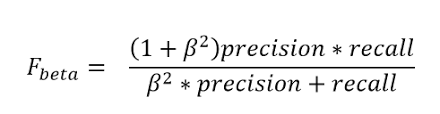->When ever if we want to reduce both FP & FN  use β=1.It is also called as F1 Score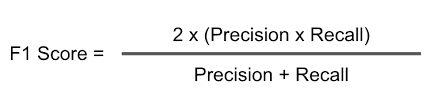--> Whenever FP is more important to reduce use β=0.5
• F0.5-Measure = ((1 + 0.5^2) * Precision * Recall) / (0.5^2 * Precision + Recall)
• F0.5-Measure = (1.25 * Precision * Recall) / (0.25 * Precision + Recall)
-->when ever FN is more important to reduce use β=2
• F2-Measure = ((1 + 2^2) * Precision * Recall) / (2^2 * Precision + Recall)
• F2-Measure = (5 * Precision * Recall) / (4 * Precision + Recall)
CO-VARIANCE:-
Covariance is nothing but the measure of the relationship between two random variables.
EX:- Height & weight of a person i.e. if the height of a person increases, the weight of that person also increases
-->If one variable is increasing & another variable also increasing its value means we can those two variables have positive co-variance.
-->If one variable is increasing & another variable is decreasing means we can say those two variables have negative co-variance.
-->The major disadvantage of covariance is covariance says whether variables are positively correlated or negatively correlated but as the covariance value is not limited to certain limits we cannot say how much two variables are positively co-related or how much negatively co-related.
Pearson correlation coefficient:-

Pearson correlation coefficient basically restricts the value between -1 to 1. The more towards +1 means more positively correlated. The near value towards -1 means negatively correlated.

From the above graph, we can say that
1. If all points fall on the same line & line moves downwards we can say it is the perfect example of negative correlation.ρ=-1
2. If all the points fall around the line and the line is decreasing means we can say it is also a negative correlation. The value of the Pearson correlation lies between -1 to 0.
3. If the point lies around the line & if the line moves upwards then we say it is a positive correlation. The value of the Pearson correlation lies between 0 to +1.
4. If the point lies the line & if the line moves upwards then we say it as a perfectly positive correlation. The value of the Pearson correlation is +1.
5. If the values are randomly distributed then we say that it has no co-relation. The value of correlation is 0.
-->Pearson correlation coefficient catches the linear property of variables very well.
Spearman's rank correlation coefficient:-
Spearman's rank correlation catches the nonlinearity property of variables also. In order to obtain this Spearman's rank correlation uses the rank of the variable.
-->Generally rank is calculated as above i.e. for Rank of IQ, Rank IQ=1 for a higher value of IQ similarly this process continues. In this way based on the value present in the column Rank is calculated and substituted in the below formula
Log-normal distribution: -
If y follows the lognormal distribution, then log(y) follows the Normal Distribution.
Bernoulli's Distribution:-
Bernoulli Distribution has two outcomes either 0 or 1.

Three examples of Bernoulli distribution:andandandBinominal Distribution:-
Binominal Distribution is the combination of multiple Bernoulli Distributions.
Power Law Distribution:-
Power law distribution is also known as the 80-20 Rule.
An example of Power Law distribution is wealth distribution i.e. 80% of the wealth is present in 20% of the population. The remaining 20% of wealth is present the 80% population
-->If we draw a graph of wealth distribution it looks like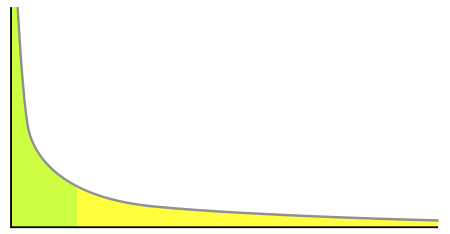The above graph is the example power-law graph, to the right has a long tail and to the left, it has a small tail. It is also known as the 80–20 rule.

Central Limit Theorem:-
Taking the entire population into consideration to draw conclusions is not possible because of time, and huge data. So, we generally consider a small amount of sample data, but taking the sample & performing the operations on the sample, and assuming the result will be applicable to the entire population data is wrong.
So, according to the central limit theorem, we will collect multiple samples of small size, then we perform certain operations on multiple sample data that we want to do. Then maximum or mean of multiple sample data results are taken into consideration by assuming this result will be applicable to population data.
For example, if you want to calculate the mean of the population(N), multiple samples(n1,n1,..) of data are collected, and then the mean is calculated on the sample data that we took. Finally, the mean of the sample means is taken into consideration as Population Mean.